﻿ 吸油烟机用多翼叶叶轮动平衡误差分析 Analysis Error on Dynamic Balance of Multi-Blade Impeller in the Range Hood

Mechanical Engineering and Technology
Vol. 08  No. 05 ( 2019 ), Article ID: 32777 , 7 pages
10.12677/MET.2019.85049

Analysis Error on Dynamic Balance of Multi-Blade Impeller in the Range Hood

Chengjie Hu1,2, Bin Li2, Ruyi Ji3, Xiaotie Zhang2, Libo He2

1School of mechanical and power engineering Tongji University, Shanghai

2Key Laboratory of Healthy & Intelligent Kitchen System Integration, Ningbo Zhejiang

3Industry Shanghai Lanya Petrochemical Equipment Inspection Institute Co. Ltd., Shanghai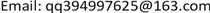Received: Oct. 10th, 2019; accepted: Oct. 24th, 2019; published: Oct. 31st, 2019ABSTRACT

In this paper, aiming at the problem of large fluctuation of the test value when the dynamic unbalanced mass of multi-blade impeller used by the range hoods are repeatedly disassembled and measured, the main factors are summed up by the combination of calculation and experiment, and the results are compared with the experimental data to verify the accuracy of the theoretical analysis, and thus reasonably explain the phenomenon where the test value will have a greater fluctuation when repeatedly disassembling and measuring the dynamic unbalanced mass of multi-blade impeller. Through the analysis and summary, we can add some design basis for designing the structure of the multi-winged impeller, but also provide the research ideas of the similar problem for other researchers and provide the reference conclusion for the similar product designer as well as some thinking for the similar product quality sampling.

Keywords:Impeller, Dynamic Imbalance Quality, Repeated Disassembly, Error Analysis

1同济大学机械与能源工程学院，上海

2浙江省健康智慧厨房系统集成重点实验室，浙江 宁波

3机械工业上海蓝亚石化设备检测所有限公司，上海1. 引言

2. 动不平衡质量重复测试数据波动的理论分析

2.1. 动平衡机的测试原理

$\left\{\begin{array}{l}{F}_{L}={N}_{L}+\frac{1}{B}\left(A{N}_{L}+C{N}_{R}\right)={m}_{1}\omega {r}^{2}\\ {F}_{R}={N}_{R}+\frac{1}{B}\left(A{N}_{L}+C{N}_{R}\right)={m}_{2}\omega {r}^{2}\end{array}$ (1)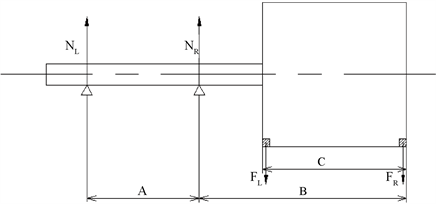Figure 1. Dynamic balance machine support solution

$x\left(t\right)={A}_{0}{A}_{1}\left(\omega \right)\mathrm{cos}\left(\omega t+{\phi }_{0}+\phi \left(\omega \right)\right)$ (2)

2.2. 双面动不平衡质量的简化计算

${m}_{i}=\frac{{M}_{i}e}{r}$ (3)

${M}_{i}$ —表示第i配平面上的叶轮转子的偏心质量(mg)；

e—表示叶轮转子整体偏心距(mm)；

${m}_{i}$ —表示第i配平面上的需要配平的质量(mg)；

r—表示转子上放置配重片的半径位置(mm)；

$M={M}_{1}+{M}_{2}$ (4)

${M}_{1}{h}_{1}={M}_{2}{h}_{2}$ (5)

${M}_{1}=\frac{M{h}_{2}}{{h}_{1}+{h}_{2}}$ (6)

${M}_{2}=\frac{M{h}_{1}}{{h}_{1}+{h}_{2}}$ (7)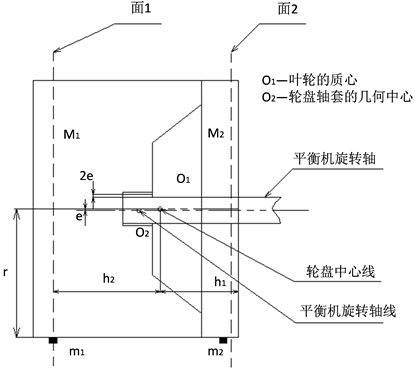Figure 2. Translation between The rotor axis and the theoretical axis of rotation

${m}_{1}=\frac{M{h}_{2}e}{\left({h}_{1}+{h}_{2}\right)r}$ (8)

${m}_{2}=\frac{M{h}_{1}e}{\left({h}_{1}+{h}_{2}\right)r}$ (9)

2.3. 孔轴极限位置引起的动不平衡量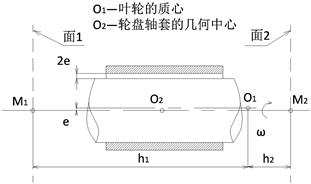Figure 3. Translation between shaft axis and hole axis

$e=\frac{轴的上偏差-孔的下偏差}{2}$

$e\approx {\text{O}}_{\text{1}}\text{A}=\frac{\text{CD}×{\text{O}}_{\text{2}}\text{A}}{\text{BD}}\approx \frac{\left(轴的上偏差-孔的下偏差\right)×{\text{O}}_{\text{2}}\text{A}}{\text{BD}}$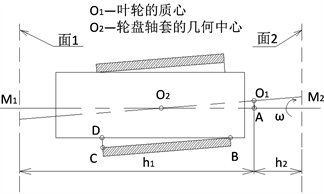Figure 4. Intersecting between shaft axis and hole axisTable 2. Dynamic imbalance of the limit position between shaft axis and hole axis

3. 实验验证和结论分析Table 3. Schenk HV2-10 dynamic balancing machine parameters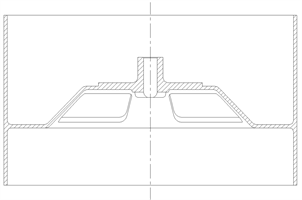Figure 5. Imitation impeller rotorTable 4. Imitation impeller rotor dynamic unbalance test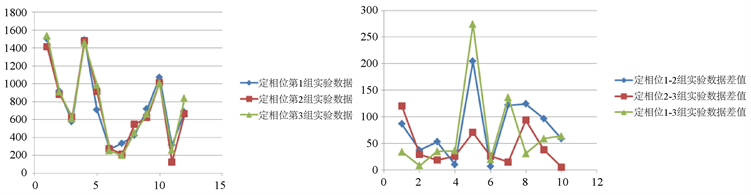Figure 6. Fixed phase dynamic imbalance test data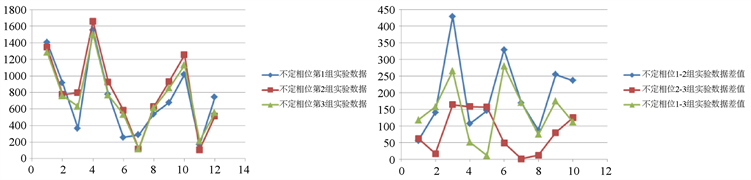Figure 7. Uncertain phase dynamic imbalance test data

4. 结论

Analysis Error on Dynamic Balance of Multi-Blade Impeller in the Range Hood[J]. 机械工程与技术, 2019, 08(05): 423-429. https://doi.org/10.12677/MET.2019.85049

1. 1. 诸永定, 易洪斌, 等. GB/T 17713 2011吸油烟机[S]. 北京: 中国标准出版社, 2012.

2. 2. 刘小民, 王星, 汤虎, 等. 我国吸油烟机性能改进技术研究进展[J]. 轻工机械, 2011, 29(3): 122-127.

3. 3. 金清肃, 郭聚东, 等. 大型引风机叶轮的动平衡问题及对策[J]. 风机技术, 2003(2): 21-22.

4. 4. 王学良. 动平衡对离心压缩机影响的分析与对策[J]. 风机技术, 2000(6): 56-57.

5. 5. 三轮修三, 下村玄. 旋转机械的平衡[M]. 北京: 机械工业出版社, 1992: 82-100.

6. 6. 宋菊平, 秦如, 等. 动不平衡测量电路频率特性原位补偿技术研究[J]. 仪表仪器学报, 2017, 38(5): 1143-1149.

7. 7. 郭天浩, 丁力. 基于Goertzel算法的转子动不平衡测量方法[J]. 测控技术, 2019, 38(7): 1143-1149.

8. 8. 魏巍, 张海鹏, 谈演. 基于M估计的转子不平衡量的识别[J]. 科技创新与应用, 2018(6): 87-89.

9. 9. Wang, A.M., Cheng, X.H., Meng, G.Y., et al. (2017) Dynamic Analysis and Numerical Experiments for Balancing of the Continuous Single-Disc and Single-Span Rotor-Bearing System. Mechanical Systems and Signal Processing, 86, 151-176. https://doi.org/10.1016/j.ymssp.2016.09.034

10. 10. 商景泰. 通风机手册[M]. 北京: 机械工业出版社, 1994: 296-307.

11. 11. 赵钧. 气浮立式硬支承动平衡机研究[J]. 中国机械工程, 2006, 17(5): 481-484.

12. 12. 陈剑飞, 梁睦, 等. 双面立式动平衡机理论分析和应用[J]. 纺织学报, 2005, 26(4): 83-85.

13. 13. Parkinson, A.G. (1991) Balancing of Rotating Machinery. Proceedings of the Institution of Mechanical Engineers, Part C: Mechanical Engineering Science, 205, 53-56. https://doi.org/10.1243/PIME_PROC_1991_205_091_02

14. 14. 余先涛. 软支承刚性转子动平衡测试系统设计[J]. 机械电子, 2000(3): 44-46.